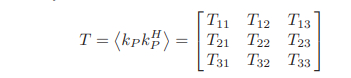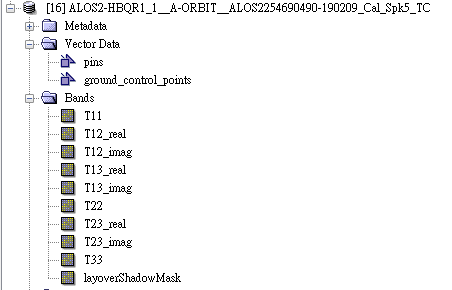# Composition and Units of Coherency Matrix Elements

Dear all,

I have confused about the composition and units of coherency matrix elements.

Figure one is the coherency matrix and figure two are outputs of T3 produced by ESA SNAP.My first question is the T12 composed of T12_real and T12_image?

T12 = T12_real + T12_image

or

T12 = T12_real (or T12_image)

And the second question is about the units of the coherency matrix.
Figures three to five are units of T11, T12_real and T12_image, they are different.

Because I want to calculate Deorientation, how to convert their units into the same unit (intensity)?